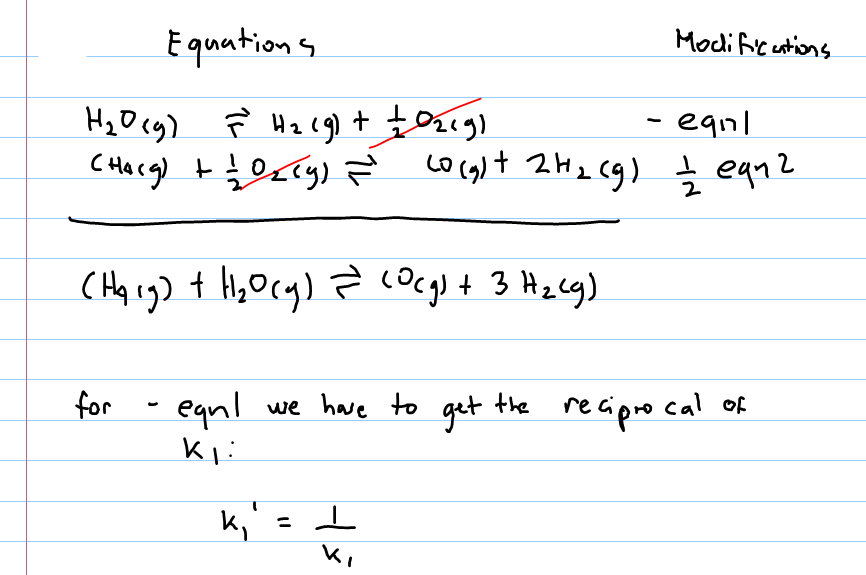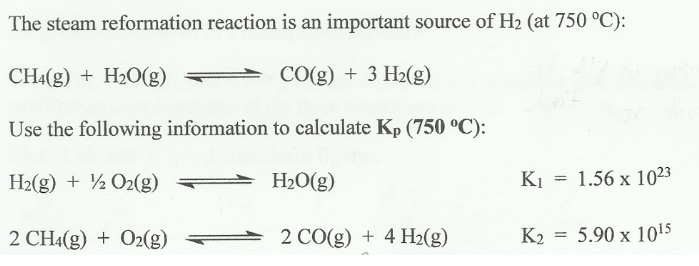# Problem: The steam reformation reaction is an important source of H2 (at 750°C): CH4 (g) + H2O (g) ⇌ CO (g) + 3H2 (g) Use the following information to calculate Kp (750°C): H2 (g) + 1/2O2 (g) ⇌ H2O (g)                        K1 = 1.56 x 1023 2CH4 (g) + O2 (g) ⇌ 2CO (g) + 4H2 (g)        K2 = 5.90 x 1015

###### FREE Expert Solution93% (71 ratings)###### Problem Details

The steam reformation reaction is an important source of H2 (at 750°C):

CH4 (g) + H2O (g) ⇌ CO (g) + 3H2 (g)

Use the following information to calculate Kp (750°C):

H2 (g) + 1/2O2 (g) ⇌ H2O (g)                        K= 1.56 x 1023

2CH4 (g) + O2 (g) ⇌ 2CO (g) + 4H2 (g)        K2 = 5.90 x 1015